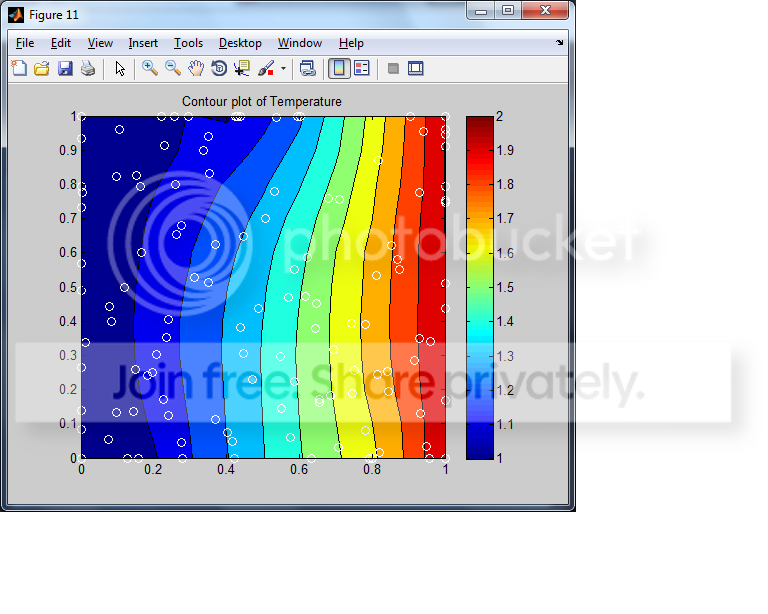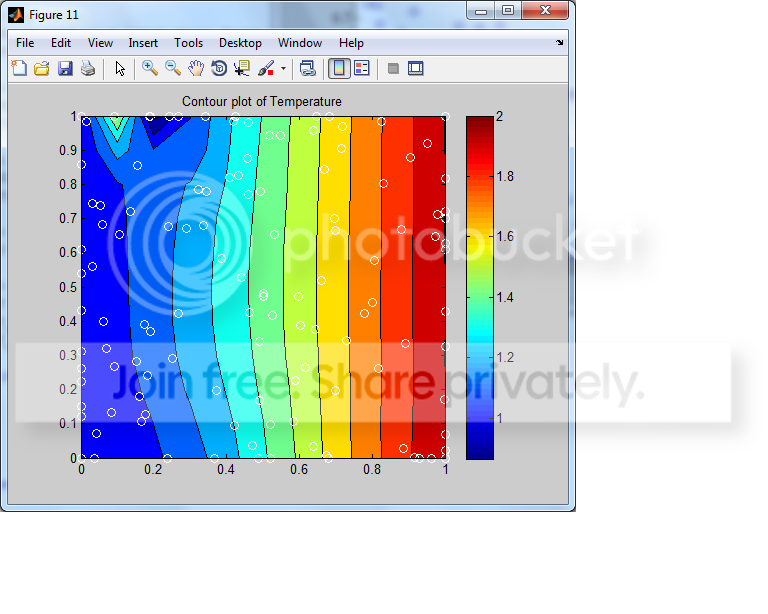1.Coding Error Help

Hey all,

I received a code from my professor using a meshless mesh. It's a simple problem but there is a problem. It is getting close to what we need it to do but its not quite there.

Spoiler: first run throughSpoiler: second run throughThe picture shows a 2-D linear temperature plot. The problem being is that the contour plot should show straight lines. Even if I increase the number of points, the problem exists, though not as bad as above.

The Code: Not very long. I cut out some of it that wasn't applicable. I almost want to say it seems like a boundary error somewhere.
Spoiler

% Test meshless method for Steady State Heat Conduction Example

% Clear workspace:
clc
close all
clear all

% Specify random or structured grids:
random_grids = 1; % 1 = random, 2 = structured

length_x = 1.0;
length_y = 1.0;

% Provide user defined values for structured grids (for grids =2):
dx = 0.04; %1/25
dy = 0.04; %1/25

% Specify parameters for random grid generation (for grids = 1):
num_avg_spanwise_grids = 11;

temp_left_wall = 1;
temp_right_wall = 2;

if random_grids == 1

dx_avg = length_x/(num_avg_spanwise_grids-1); %=0.025
dy_avg = length_y/(num_avg_spanwise_grids-1); %=0.025

dx = dx_avg; %=0.025
dy = dy_avg; %=0.025

% Define number of nodes
num_x_nodes = length_x/dx+1; %=41 1x1 matrix
num_y_nodes = length_y/dy+1; %=41 1x1 matrix
total_nodes = num_x_nodes*num_y_nodes; %=1681 1x1 matrix
grids = 1:total_nodes; %1x1681 matrix

%Define 1681x1681 matrix and 1681x1 matrix
Z = zeros(total_nodes,total_nodes); %1681x1681 matrix
Z1 = zeros(total_nodes,1); %1681x1 matrix

% Define shape parameter value based on given grid domain:
%c = dx; % okay 0.025
%c = 0.815*sqrt(dx^2+dy^2); % moderate 0.0281
%c = 1.25*sqrt(dx^2+dy^2)/sqrt(total_nodes); % bad .0010779066786
c = 2/sqrt(total_nodes); % great 0.04878
c=0.1

% Preallocate matrices for speed:
coord_x = Z1; %1681x1 matrix
coord_y = Z1; %1681x1 matrix

K = Z; %1681x1681 matrix
F = Z1; %1681x1 matrix

delxsq = Z; %1681x1681 matrix
delysq = Z; %1681x1681 matrix
delrsq = Z; %1681x1681 matrix

total_shape_op = Z; %1681x1681 matrix
total_shape_deriv_x_op = Z; %1681x1681 matrix
total_shape_deriv_y_op = Z; %1681x1681 matrix
total_shape_laplacian_op = Z; %1681x1681 matrix

% Define all grid sets:
all_points = 1:total_nodes; %1x1681 matrix
bottom_wall = 1:num_x_nodes; %1x41 matrix
top_wall = (num_y_nodes-1)*num_x_nodes+1:total_nodes; %1x41 matrix
left_wall = 1:num_x_nodes:((num_y_nodes-1)*num_x_nodes+1); %1x41 matrix
right_wall = num_x_nodes:num_x_nodes:total_nodes; %1x41 matrix
interior_points = setdiff(all_points,[bottom_wall,top_wall,left_wall,right_wall]); %1x1521

%left_wall=left_wall' %41x1 matrix
%right_wall=right_wall' %41x1 matrix

% Randomize all points:
for L = all_points % 1:total_nodes 1x1681 matrix
coord_x(L) = rand*length_x;
coord_y(L) = rand*length_y;
end

% Specify coordinate restrictions on walls (which will result in
% correct edge and corner coordinates since points overlap properly)
% Walls:
coord_y(bottom_wall) = 0;
coord_y(top_wall) = length_y;

coord_x(left_wall) = 0;
coord_x(right_wall) = length_x;

% Plot grid coordinate locations:
figure(1)
plot(coord_x,coord_y,'bo')
title('Grid coordinate locations')

end

% Create completely sampled shape operator:
for i = 1:total_nodes
for j = 1:total_nodes
delxsq(i,j) = (coord_x(i)-coord_x(j))^2;
delysq(i,j) = (coord_y(i)-coord_y(j))^2;
delrsq(i,j) = delxsq(i,j)+delysq(i,j);

total_shape_op(i,j) = sqrt(delrsq(i,j)+c^2);
total_shape_deriv_x_op(i,j) = (coord_x(i)-coord_x(j))/(delrsq(i,j)+c^2)^(1/2);
total_shape_deriv_y_op(i,j) = (coord_y(i)-coord_y(j))/(delrsq(i,j)+c^2)^(1/2);
total_shape_laplacian_op(i,j) = (delrsq(i,j)+2*c^2)/(delrsq(i,j)+c^2)^(3/2);
end
end

% Apply interior domain conditions:
K(interior_points,:) = total_shape_laplacian_op(interior_points,:);
F(interior_points,1) = 0;

% Apply boundary domain conditions:

% Bottom wall:
K(bottom_wall,:) = total_shape_deriv_y_op(bottom_wall,:);
F(bottom_wall,1) = 0; % Value of derivative on bottom wall

% Top wall:
K(top_wall,:) = total_shape_deriv_y_op(top_wall,:);
F(top_wall,1) = 0; % Value of derivative on top wall

% Left wall:
K(left_wall,:) = total_shape_op(left_wall,:);
F(left_wall,1) = temp_left_wall; % Value of temperature on left wall

% Right wall:
K(right_wall,:) = total_shape_op(right_wall,:);
F(right_wall,1) = temp_right_wall; % Value of temperature on right wall

% Solve system of equations:
U = K\F;

% Derive actual temperature and temperature derivative values:
T = total_shape_op*U;
T_y_deriv = total_shape_deriv_y_op*U;
T_laplacian = total_shape_laplacian_op*U;

if random_grids == 1

[X,Y] = meshgrid([0:(dx_avg):length_y],[0:(dy_avg):length_y]);
T_plot = griddata(coord_x,coord_y,T,X,Y,'v4');
F_2D_interp = TriScatteredInterp(coord_x, coord_y, T);
T_plot = F_2D_interp(X,Y);

end

% Plot results and boundary conditions to test accuracy
figure(11)
contourf(X,Y,T_plot)
title('Contour plot of Temperature')
hold on
plot(coord_x,coord_y,'wo')
colorbar
hold off

figure(21)
surf(X,Y,T_plot)
title('Surface plot of Temperature')Reply With Quote

2.Re: Coding Error Help

Hello, Deathslayer.

I'm a little bit puzzled because I still don't quite understand what you're trying to do. If I understand this correctly, you're generating random points and then plotting lines through them, the whole producing a set of contours. Is that correct?

Of course, random points don't line up in neat straight lines -- that is artificial order that must be imposed by the program. So I think you need a line of best fit , if you're looking to get straight lines out of this. Which is, the data won't line up naturally in straight lines and simply interpolating will give you the behavior you're seeing. If you want straight lines, you're going to need to first collect related points together into sets, then for each set generate a best-fit line to approximate the relationship between the different points in an individual set. One set, one line.

Those are my suggestions. I'm sorry if it's not more helpful, but my time is limited, I'm afraid :).

Respectfully,

Brian P.Reply With Quote

3.Re: Coding Error Help

Why are the lines supposed to be straight? If I'm remembering what a contour plot is correctly, straight lines have nothing to do with it.Reply With QuotePosting Permissions

• You may not post new threads
• You may not post replies
• You may not post attachments
• You may not edit your posts
•